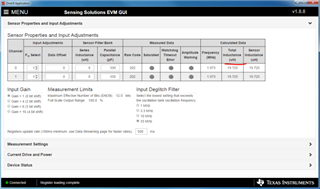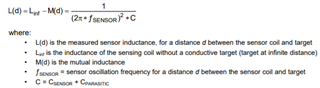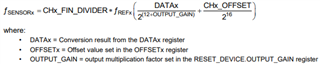If you have a related question, please click the "Ask a related question" button in the top right corner. The newly created question will be automatically linked to this question.

# LDC1312EVM: Getting Consistent Results

Part Number: LDC1312EVM

Hello,

We are using the LDC1312EVM and having difficulty getting consistent data from the GUI when we repeat tests at a later time.

Maybe it has to do with the auto calculated Total Inductance parameter, but I’m not sure how that value is generated and therefor am struggling to debug my issue.

Here is the screen capture below.  Thank you for your help.  Keith• Hello,

The "Total Inductance" parameter is the calculated inductance based on the sensor data. If you have a series inductance, the offset from that will be subtracted from the total inductance so that your sensor inductance doesn't include the series inductor.

The inductance value is calculated based on the sensor frequency and known capacitance.The frequency of the sensor is calculated based on the data output of the device, the frequency divider, output gain, and any offset:As for inconsistency between measurements, environmental factors such as the temperature can have an impact on the sensor frequency. One test you can do to verify consistency is to measure the sensor frequency with an oscilloscope and make sure that is consistent between tests. If that is consistent, then double check that register settings are the same so that the calculations match.

Best Regards,

Justin Beigel